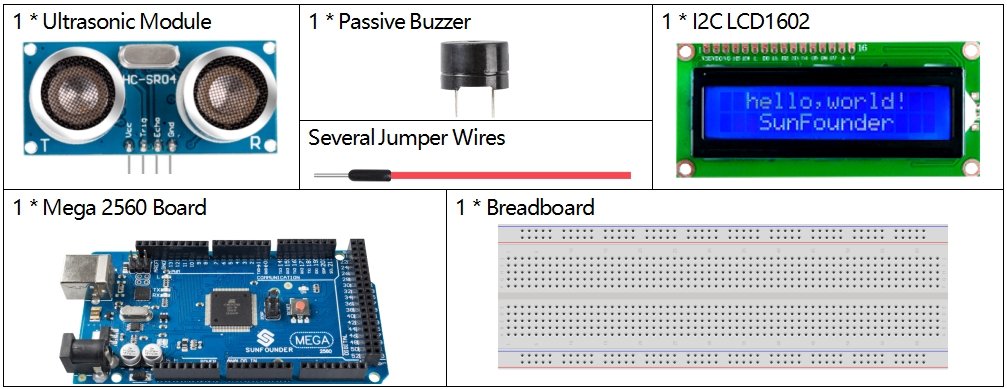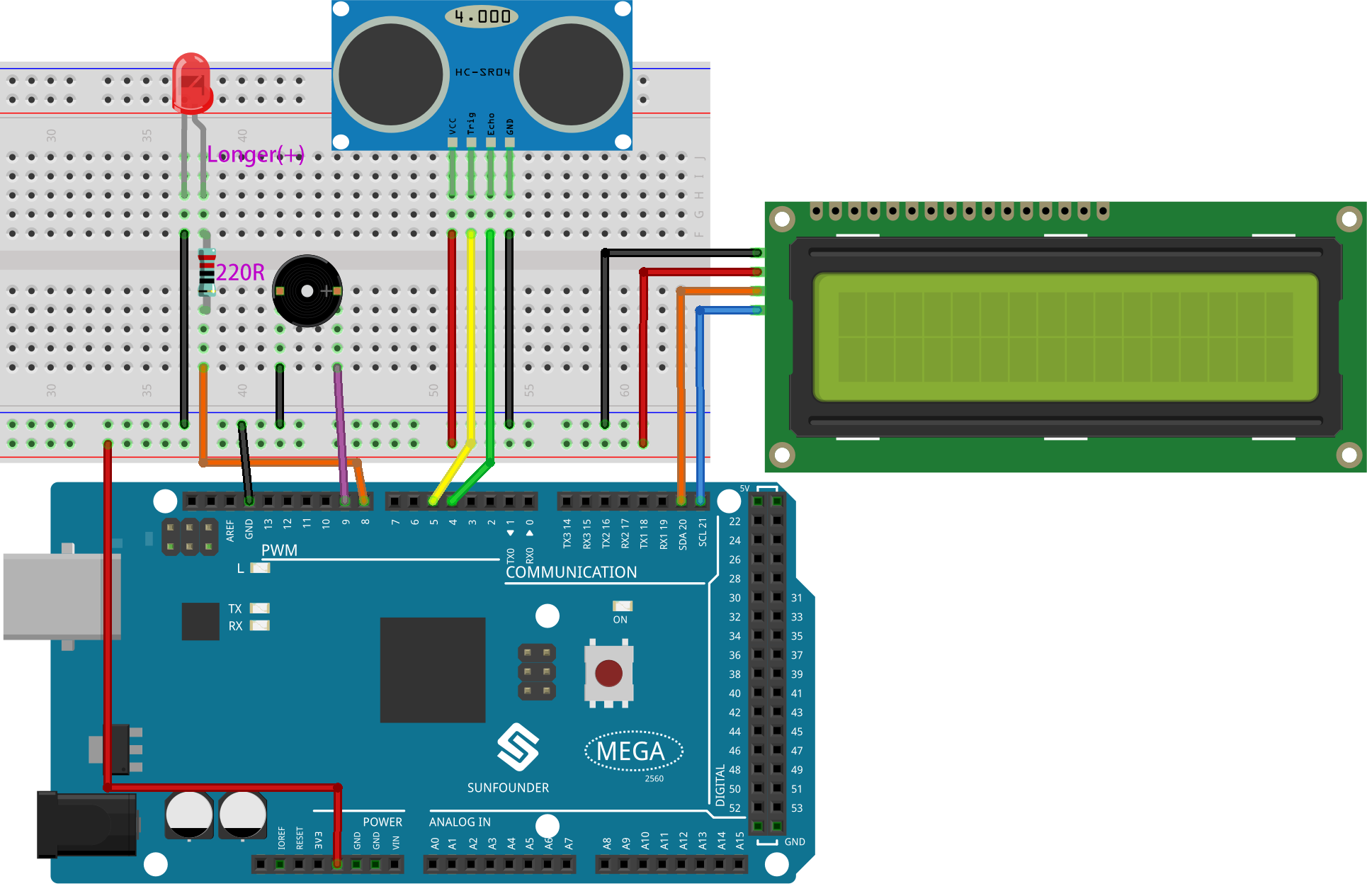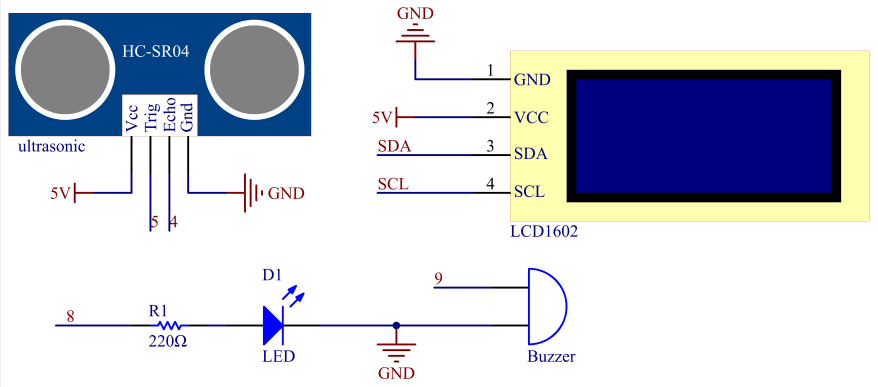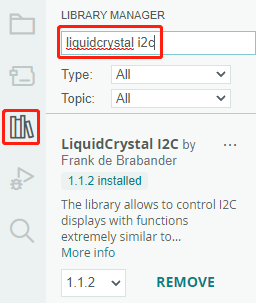# 3.1 Reversing Aid¶

## Overview¶

With the development of science and technology, a lot of high-tech products have been installed in cars, among which the reversing assist system is one of them. Here we use ultrasonic sensors, LCD, LED and buzzer to make a simple ultrasonic reversing assist system.

## Components Required¶## Fritzing Circuit¶

In this example, the wiring is shown below.## Schematic Diagram¶## Code¶

Note

• You can open the file `3.1_reversingAid.ino` under the path of `sunfounder_vincent_kit_for_arduino\code\3.1_reversingAid` directly.

• The `LiquidCrystal I2C` library is used here, you can install it from the Library Manager.## Example Explanation¶

This code helps us create a simple distance measuring device that can measure the distance between objects and provide feedback through an LCD display and a buzzer.

The `loop()` function contains the main logic of the program and runs continuously. Let’s take a closer look at the `loop()` function.

1. Loop to read distance and update parameters

In the `loop`, the code first reads the distance measured by the ultrasonic module and updates the interval parameter based on the distance.

```// Update the distance

// Update intervals based on distance
if (distance <= 10) {
intervals = 300;
} else if (distance <= 20) {
intervals = 500;
} else if (distance <= 50) {
intervals = 1000;
} else {
intervals = 2000;
}
```
2. Check if it’s time to beep

The code calculates the difference between the current time and the previous beep time, and if the difference is greater than or equal to the interval time, it triggers the buzzer and updates the previous beep time.

```unsigned long currentMillis = millis();
if (currentMillis - previousMillis >= intervals) {
Serial.println("Beeping!");
beep();
previousMillis = currentMillis;
}
```
3. Update LCD display

The code clears the LCD display and then displays “Dis:” and the current distance in centimeters on the first line.

```lcd.clear();
lcd.setCursor(0, 0);
lcd.print("Dis: ");
lcd.print(distance);
lcd.print(" cm");

delay(100);
```